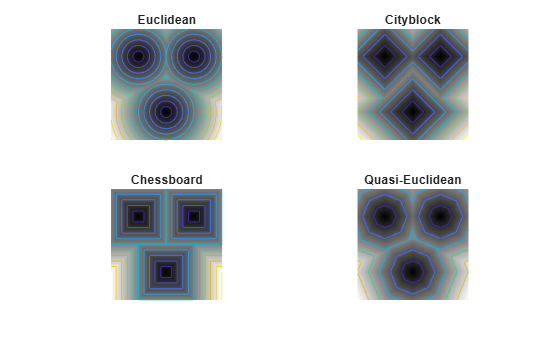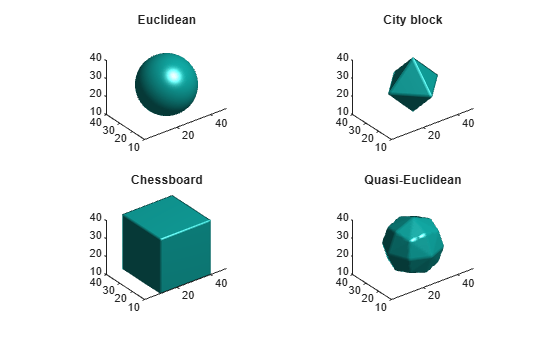# bwdist

Distance transform of binary image

## Syntax

``D = bwdist(BW)``
``[D,idx] = bwdist(BW)``
``[D,idx] = bwdist(BW,method)``

## Description

````D = bwdist(BW)` computes the Euclidean distance transform of the binary image `BW`. For each pixel in `BW`, the distance transform assigns a number that is the distance between that pixel and the nearest nonzero pixel of `BW`.You optionally can compute the Euclidean distance transform of a 2-D binary image using a GPU (requires Parallel Computing Toolbox™).```
````[D,idx] = bwdist(BW)` also computes the closest-pixel map in the form of an index array, `idx`. Each element of `idx` contains the linear index of the nearest nonzero pixel of `BW`. The closest-pixel map is also called the feature map, feature transform, or nearest-neighbor transform.```
````[D,idx] = bwdist(BW,method)` computes the distance transform using an alternate distance metric, specified by `method`.```

## Examples

collapse all

This example shows how to compute the Euclidean distance transform of a binary image, and the closest-pixel map of the image.

Create a binary image.

```bw = zeros(5,5); bw(2,2) = 1; bw(4,4) = 1```
```bw = 5×5 0 0 0 0 0 0 1 0 0 0 0 0 0 0 0 0 0 0 1 0 0 0 0 0 0 ```

Calculate the distance transform.

`[D,IDX] = bwdist(bw)`
```D = 5x5 single matrix 1.4142 1.0000 1.4142 2.2361 3.1623 1.0000 0 1.0000 2.0000 2.2361 1.4142 1.0000 1.4142 1.0000 1.4142 2.2361 2.0000 1.0000 0 1.0000 3.1623 2.2361 1.4142 1.0000 1.4142 ```
```IDX = 5x5 uint32 matrix 7 7 7 7 7 7 7 7 7 19 7 7 7 19 19 7 7 19 19 19 7 19 19 19 19 ```

In the nearest-neighbor matrix `IDX` the values 7 and 19 represent the position of the nonzero elements using linear matrix indexing. If a pixel contains a 7, its closest nonzero neighbor is at linear position 7.

This example shows how to compare the 2-D distance transforms for supported distance methods. In the figure, note how the quasi-Euclidean distance transform best approximates the circular shape achieved by the Euclidean distance method.

```bw = zeros(200,200); bw(50,50) = 1; bw(50,150) = 1; bw(150,100) = 1; D1 = bwdist(bw,'euclidean'); D2 = bwdist(bw,'cityblock'); D3 = bwdist(bw,'chessboard'); D4 = bwdist(bw,'quasi-euclidean'); RGB1 = repmat(rescale(D1), [1 1 3]); RGB2 = repmat(rescale(D2), [1 1 3]); RGB3 = repmat(rescale(D3), [1 1 3]); RGB4 = repmat(rescale(D4), [1 1 3]); figure subplot(2,2,1), imshow(RGB1), title('Euclidean') hold on, imcontour(D1) subplot(2,2,2), imshow(RGB2), title('City block') hold on, imcontour(D2) subplot(2,2,3), imshow(RGB3), title('Chessboard') hold on, imcontour(D3) subplot(2,2,4), imshow(RGB4), title('Quasi-Euclidean') hold on, imcontour(D4)```This example shows how to compare isosurface plots for the distance transforms of a 3-D image containing a single nonzero pixel in the center.

```bw = zeros(50,50,50); bw(25,25,25) = 1; D1 = bwdist(bw); D2 = bwdist(bw,'cityblock'); D3 = bwdist(bw,'chessboard'); D4 = bwdist(bw,'quasi-euclidean'); figure subplot(2,2,1), isosurface(D1,15), axis equal, view(3) camlight, lighting gouraud, title('Euclidean') subplot(2,2,2), isosurface(D2,15), axis equal, view(3) camlight, lighting gouraud, title('City block') subplot(2,2,3), isosurface(D3,15), axis equal, view(3) camlight, lighting gouraud, title('Chessboard') subplot(2,2,4), isosurface(D4,15), axis equal, view(3) camlight, lighting gouraud, title('Quasi-Euclidean')```## Input Arguments

collapse all

Binary image, specified as a numeric or logical array of any dimension. For numeric input, any nonzero pixels are considered to be `1` (`true`).

Data Types: `single` | `double` | `int8` | `int16` | `int32` | `int64` | `uint8` | `uint16` | `uint32` | `uint64` | `logical`

Distance metric, specified as one of the these values.

Method

Description

`'chessboard'`

In 2-D, the chessboard distance between (x1,y1) and (x2,y2) is

max(│x1x2│,│y1y2│).

`'cityblock'`

In 2-D, the cityblock distance between (x1,y1) and (x2,y2) is

x1x2│ + │y1y2

`'euclidean'`

In 2-D, the Euclidean distance between (x1,y1) and (x2,y2) is

`$\sqrt{{\left({x}_{1}-{x}_{2}\right)}^{2}+{\left({y}_{1}-{y}_{2}\right)}^{2}}.$`

`'quasi-euclidean'`

In 2-D, the quasi-Euclidean distance between (x1,y1) and (x2,y2) is

Only the `'euclidean'` method is supported on a GPU.

Data Types: `char` | `string`

## Output Arguments

collapse all

Distance, returned as a numeric array of the same size as `BW`. The value of each element is the distance between that pixel and the nearest nonzero pixel in `BW`, as defined by the distance metric, `method`.

Data Types: `single`

Index array, returned as a numeric array of the same size as `BW`. Each element of `idx` contains the linear index of the nearest nonzero pixel of `BW`. The class of `idx` depends on the number of elements in the input image, and is determined as follows.

ClassRange
`'uint32'``numel(BW)` <= 232 − 1
`'uint64'``numel(BW)` >= 232

Data Types: `uint32` | `uint64`

## Tips

• `bwdist` uses fast algorithms to compute the true Euclidean distance transform, especially in the 2-D case. The other methods are provided primarily for pedagogical reasons. However, the alternative distance transforms are sometimes significantly faster for multidimensional input images, particularly those that have many nonzero elements.

• The function `bwdist` changed in version 6.4 (R2009b). Previous versions of the Image Processing Toolbox used different algorithms for computing the Euclidean distance transform and the associated label matrix. If you need the same results produced by the previous implementation, use the function `bwdist_old`.

## Algorithms

• For Euclidean distance transforms, `bwdist` uses the fast algorithm. 

• For cityblock, chessboard, and quasi-Euclidean distance transforms, `bwdist` uses the two-pass, sequential scanning algorithm. 

• The different distance measures are achieved by using different sets of weights in the scans, as described in .

 Maurer, Calvin, Rensheng Qi, and Vijay Raghavan, "A Linear Time Algorithm for Computing Exact Euclidean Distance Transforms of Binary Images in Arbitrary Dimensions," IEEE Transactions on Pattern Analysis and Machine Intelligence, Vol. 25, No. 2, February 2003, pp. 265-270.

 Rosenfeld, Azriel and John Pfaltz, "Sequential operations in digital picture processing," Journal of the Association for Computing Machinery, Vol. 13, No. 4, 1966, pp. 471-494.

 Paglieroni, David, "Distance Transforms: Properties and Machine Vision Applications," Computer Vision, Graphics, and Image Processing: Graphical Models and Image Processing, Vol. 54, No. 1, January 1992, pp. 57-58.